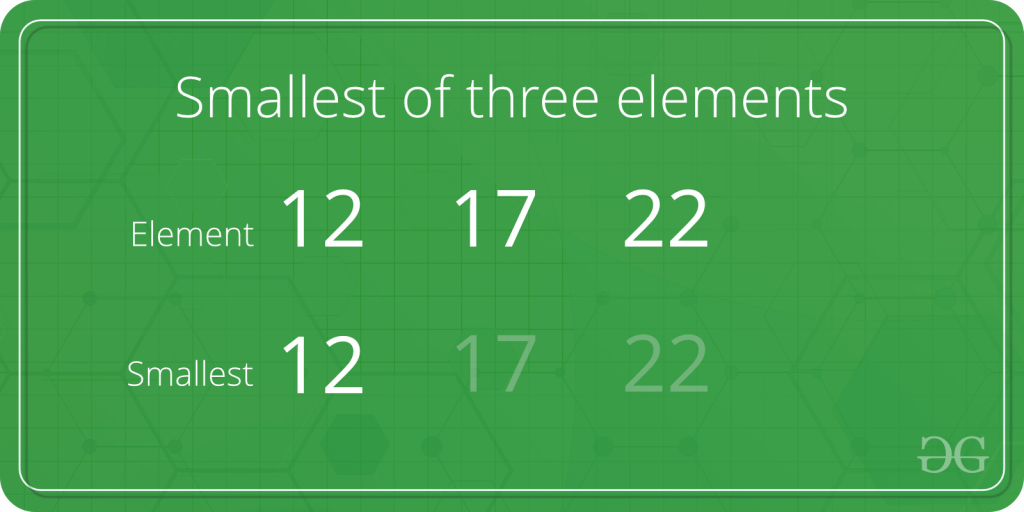Open in App
Not now

# Program to find the smallest element among three elements

• Difficulty Level : Basic
• Last Updated : 16 Dec, 2022

Given three numbers. The task is to find the smallest among the given three numbers.Examples:

```Input: first = 15, second = 16, third = 10
Output: 10

Input: first = 5, second = 3, third = 6
Output: 3```

Approach:

1. Check if the first element is smaller than or equal to second and third. If yes then print it.
2. Else if check if the second element is smaller than or equal to first and third. If yes then print it.
3. Else third is the smallest element and print it.

Below is the implementation of the above approach:

## C++

 `// C++ implementation to find` `// the smallest of three elements` `#include ` `using` `namespace` `std;`   `int` `main()` `{` `    ``int` `a = 5, b = 7, c = 10;`   `    ``if` `(a <= b && a <= c)` `        ``cout << a << ``" is the smallest"``;`   `    ``else` `if` `(b <= a && b <= c)` `        ``cout << b << ``" is the smallest"``;`   `    ``else` `        ``cout << c << ``" is the smallest"``;`   `    ``return` `0;` `}`

## C

 `// C implementation to find` `// the smallest of three elements` `#include `   `int` `main()` `{` `    ``int` `a = 5, b = 7, c = 10;`   `    ``if` `(a <= b && a <= c)` `        ``printf``(``"%d is the smallest"``,a);`   `    ``else` `if` `(b <= a && b <= c)` `        ``printf``(``"%d is the smallest"``,b);`   `    ``else` `        ``printf``(``"%d is the smallest"``,c);`   `    ``return` `0;` `}`   `// This code is contributed by kothavvsaakash.`

## Java

 `// Java implementation to find` `// the smallest of three elements`   `import` `java.io.*;`   `class` `GFG {` `  `      `    ``public` `static` `void` `main (String[] args) {` `            ``int` `a = ``5``, b = ``7``, c = ``10``;`   `    ``if` `(a <= b && a <= c)` `        ``System.out.println( a + ``" is the smallest"``);`   `    ``else` `if` `(b <= a && b <= c)` `        ``System.out.println( b + ``" is the smallest"``);`   `    ``else` `        ``System.out.println( c + ``" is the smallest"``);`   `    ``}` `}` `// This code is contributed by shs..`

## Python3

 `# Python implementation to find` `# the smallest of three elements`   `a, b, c ``=` `5``, ``7``, ``10`   `if``(a <``=` `b ``and` `a <``=` `c):` `    ``print``(a, ``"is the smallest"``)`   `elif``(b <``=` `a ``and` `b <``=` `c):` `    ``print``(b, ``"is the smallest"``)` `else``:` `    ``print``(c, ``"is the smallest"``)`   `# This code is contributed ` `# by 29AjayKumar`

## C#

 `// C# implementation to find ` `// the smallest of three elements` `using` `System;`   `class` `GFG` `{` `static` `public` `void` `Main ()` `{` `int` `a = 5, b = 7, c = 10; ` `if` `(a <= b && a <= c) ` `    ``Console.WriteLine( a + ``" is the smallest"``); `   `else` `if` `(b <= a && b <= c) ` `    ``Console.WriteLine( b + ``" is the smallest"``); `   `else` `    ``Console.WriteLine( c + ``" is the smallest"``); ` `}` `}`   `// This code is contributed by jit_t`

## PHP

 `

## Javascript

 ``

Output

`5 is the smallest`

Time complexity : O(1)
Auxiliary Space: O(1)

My Personal Notes arrow_drop_up
Related Articles